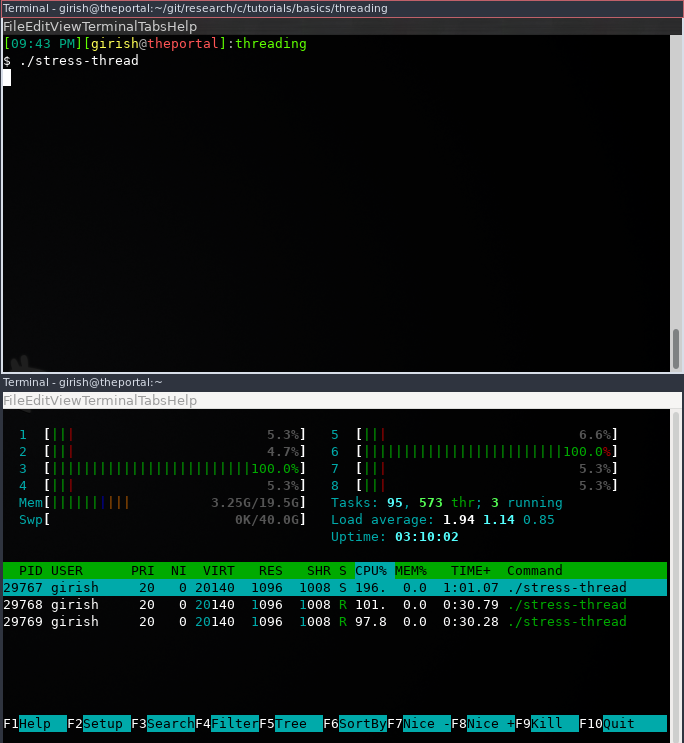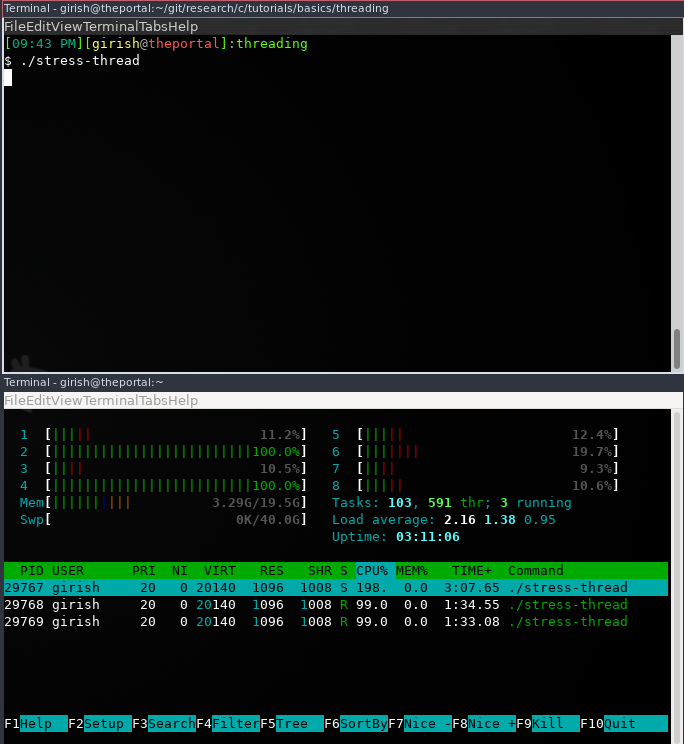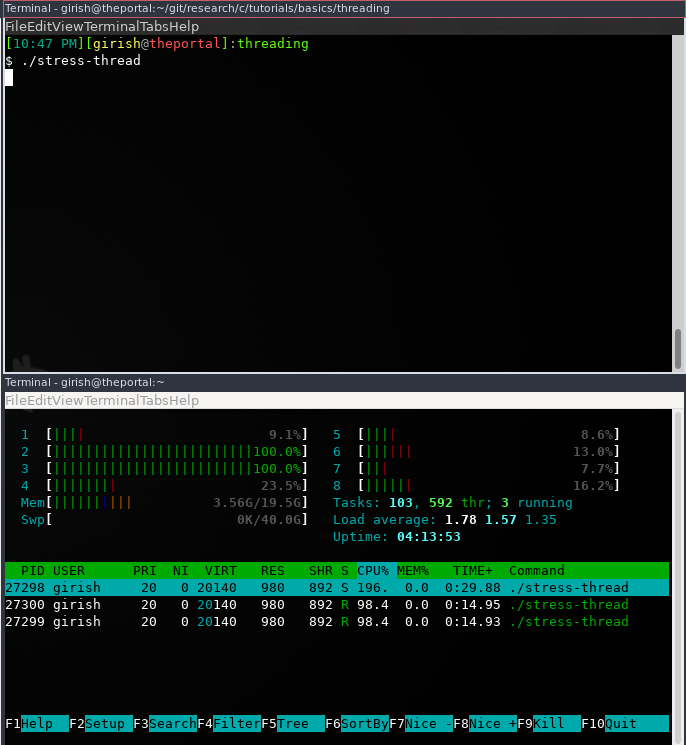# Glibc: Pthread - Setting Cpu Affinity - Linux

## CPU Affinity.

`CPU affinity` is the ability to bind process or thread to processor. It is useful when you need to run a given process on a specific `CPU`/`Core`.

This can be very usefull specifically in two situations,

• When you need to reduce cache miss rate.
• When you are running real-time or time sensitive applications.

You can set the `CPU affinity` on linux using the tool taskset. If you want to set the `CPU affinity` programatically, it can be done using sched_setaffinity. For a thread `CPU affinity` can be set using pthread_setaffinity_np and pthread_attr_setaffinity_np

In this post I’ll be covering setting `CPU affinity` for pthreads.

## How do we do that and how do we verify?

In order to set the affinity and verify that the given thread is running on the specific `CPU`.

• Get a piece of code which is CPU intensive.
• Run it in two separate threadas.
• Check in `htop` if they switch the cores.
• In the next step, get the number of available cores.
• Create a `CPUSET` with one `CPU`.
• Start the thread with affinity to that `CPUSET`.
• Observe in `htop` if the threads switch cores.

## The code.

### The CPU intensive code.

We can calculate something in an infinite loop.

``````#include <math.h>

void *cpu_intensive_function(void *args){
float x = 1.5f;
while (1)
{
x *= sin(x) / atan(x) * tanh(x) * sqrt(x);
}
}``````

``````#include <pthread.h>

int main(){

int th1_op = pthread_create(&th1, NULL, cpu_intensive_function, NULL);
int th2_op = pthread_create(&th2, NULL, cpu_intensive_function, NULL);

return 0;
}``````

When you run this code, you can observe in `htop` that the threads switch the cores.A few moments later.### Create CPUSETs.

`cpu_set_t` represents the set of `CPUs` that can be used for scheculing a thread/process on that set of `CPUs`.

``````// Create a cpuset
cpu_set_t cpuset_1;

// Clear the cpuset
CPU_ZERO(&cpuset_1);

// add cpu core 1 to the cpuset
CPU_SET(1, &cpuset_1);``````

### Set the CPU affinity.

`pthread_setaffinity_np()` takes a `thread`, length of buffer pointed to `cpu_set_t`, and a `cpu_set_t` as the arguments. It basically sets the CPU affinity mask for the given thread to the given set of `CPUs`.

``````#include <errno.h>

#define handle_error_en(en, msg)\
do { errno = en; perror(msg); exit(EXIT_FAILURE);} while (0);

// create a cpu set with core 1
cpu_set_t cpuset_1;
CPU_ZERO(&cpuset_1);
CPU_SET(1, &cpuset_1);

// create a cpu set with core 2
cpu_set_t cpuset_2;
CPU_ZERO(&cpuset_2);
CPU_SET(2, &cpuset_2);

int ret1;
int ret2;

ret1 = pthread_create(&th1, NULL, cpu_intensive_function, NULL);
ret2 = pthread_create(&th2, NULL, cpu_intensive_function, NULL);

/* Set the affinity to thread 1*/
if (s1 != 0)

/*set the affinity to thread 2*/
if (s2 != 0)

// wait for the threads to terminate.

### Putting everything together.

``````#define _GNU_SOURCE
#include <stdio.h>
#include <stdlib.h>
#include <unistd.h>
#include <math.h>
#include <errno.h>

#define handle_error_en(en, msg)\
do { errno = en; perror(msg); exit(EXIT_FAILURE);} while (0);

void *cpu_intensive_function(void *args){
float x = 1.5f;
while (1)
{
x *= sin(x) / atan(x) * tanh(x) * sqrt(x);
}
}

int main(){

// create a cpu set with core 1
cpu_set_t cpuset_1;
CPU_ZERO(&cpuset_1);
CPU_SET(1, &cpuset_1);

// create a cpu set with core 2
cpu_set_t cpuset_2;
CPU_ZERO(&cpuset_2);
CPU_SET(2, &cpuset_2);

int ret1;
int ret2;

ret1 = pthread_create(&th1, NULL, cpu_intensive_function, NULL);
ret2 = pthread_create(&th2, NULL, cpu_intensive_function, NULL);

/* Set the affinity to thread 1*/
int s1 = pthread_setaffinity_np(th1, sizeof(cpu_set_t), &cpuset_1);
if (s1 != 0)

/*set the affinity to thread 2*/
int s2 = pthread_setaffinity_np(th2, sizeof(cpu_set_t), &cpuset_2);
if (s2 != 0)